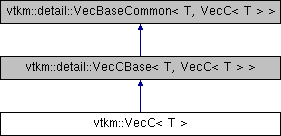VTK-m  2.0
vtkm::VecC< T > Class Template Reference

A Vec-like representation for short arrays. More...

`#include <Types.h>`

Inheritance diagram for vtkm::VecC< T >:## Public Types

using ComponentType = T

## Public Member Functions

VTKM_EXEC_CONT VecC ()

VTKM_EXEC_CONT VecC (T *array, vtkm::IdComponent size)

template<vtkm::IdComponent Size>
VTKM_EXEC_CONT VecC (vtkm::Vec< T, Size > &src)

VTKM_EXEC_CONT VecC (T &src)

VTKM_EXEC_CONT VecC (const VecC< T > &src)

const VTKM_EXEC_CONT T & operator[] (vtkm::IdComponent index) const

VTKM_EXEC_CONT T & operator[] (vtkm::IdComponent index)

VTKM_EXEC_CONT vtkm::IdComponent GetNumberOfComponents () const

VTKM_EXEC_CONT VecC< T > & operator= (const VecC< T > &src)

## Private Types

using Superclass = detail::VecCBase< T, VecC< T > >

## Private Member Functions

VTKM_STATIC_ASSERT_MSG (std::is_const< T >::value==false, "You cannot use VecC with a const type as its template argument. " "Use either const VecC or VecCConst.")

## Private Attributes

T *const Components

vtkm::IdComponent NumberOfComponents

## Detailed Description

### template<typename T> class vtkm::VecC< T >

A Vec-like representation for short arrays.

The `VecC` class takes a short array of values and provides an interface that mimics `Vec`. This provides a mechanism to treat C arrays like a `Vec`. It is useful in situations where you want to use a `Vec` but the data must come from elsewhere or in certain situations where the size cannot be determined at compile time. In particular, `Vec` objects of different sizes can potentially all be converted to a `VecC` of the same type.

Note that `VecC` holds a reference to an outside array given to it. If that array gets destroyed (for example because the source goes out of scope), the behavior becomes undefined.

You cannot use `VecC` with a const type in its template argument. For example, you cannot declare `VecC<const vtkm::Id>`. If you want a non-mutable `VecC`, the `VecCConst` class (e.g. `VecCConst<vtkm::Id>`).

## ◆ ComponentType

template<typename T >
 using vtkm::VecC< T >::ComponentType = T

## ◆ Superclass

template<typename T >
 using vtkm::VecC< T >::Superclass = detail::VecCBase >
private

## ◆ VecC() [1/5]

template<typename T >
 VTKM_EXEC_CONT vtkm::VecC< T >::VecC ( )
inline

## ◆ VecC() [2/5]

template<typename T >
 VTKM_EXEC_CONT vtkm::VecC< T >::VecC ( T * array, vtkm::IdComponent size )
inline

## ◆ VecC() [3/5]

template<typename T >
template<vtkm::IdComponent Size>
 VTKM_EXEC_CONT vtkm::VecC< T >::VecC ( vtkm::Vec< T, Size > & src )
inline

## ◆ VecC() [4/5]

template<typename T >
 VTKM_EXEC_CONT vtkm::VecC< T >::VecC ( T & src )
inlineexplicit

## ◆ VecC() [5/5]

template<typename T >
 VTKM_EXEC_CONT vtkm::VecC< T >::VecC ( const VecC< T > & src )
inline

## ◆ GetNumberOfComponents()

template<typename T >
 VTKM_EXEC_CONT vtkm::IdComponent vtkm::VecC< T >::GetNumberOfComponents ( ) const
inline

## ◆ operator=()

template<typename T >
 VTKM_EXEC_CONT VecC& vtkm::VecC< T >::operator= ( const VecC< T > & src )
inline

## ◆ operator[]() [1/2]

template<typename T >
 VTKM_EXEC_CONT T& vtkm::VecC< T >::operator[] ( vtkm::IdComponent index )
inline

## ◆ operator[]() [2/2]

template<typename T >
 const VTKM_EXEC_CONT T& vtkm::VecC< T >::operator[] ( vtkm::IdComponent index ) const
inline

## ◆ VTKM_STATIC_ASSERT_MSG()

template<typename T >
 vtkm::VecC< T >::VTKM_STATIC_ASSERT_MSG ( std::is_const< T >::value = `=false`, "You cannot use VecC< T > with a const type as its template argument. " "Use either const VecC< T > or VecCConst." )
private

## ◆ Components

template<typename T >
 T* const vtkm::VecC< T >::Components
private

## ◆ NumberOfComponents

template<typename T >
 vtkm::IdComponent vtkm::VecC< T >::NumberOfComponents
private

The documentation for this class was generated from the following file: